# UNIT HYDROGRAPHReading time: 1 minute

#### UNIT HYDROGRAPH

• Very often it is required to predict the flood hydrograph resulting from a known storm

• A large number of methods are available to solve this problem

Unit Hydrograph Method is the most popular and widely used method for predicting flood hydrograph resulting from a known storm

• First suggested by Sherman in 1932

Definition : A unit hydrograph is defined as the hydrograph of direct runoff resulting from one unit depth (1cm) of rainfall excess occurring uniformly over the basin and at a uniform rate for a specified duration (D hours)

• The term ‘unit’ refers to a unit depth of rainfall excess which is usually taken as 1cm

• The duration is used as a prefix to a specific unit hydrograph

The definition of a unit hydrograph implies the following:

1. The unit hydrograph represents the lumped response of the catchment to a unit rainfall excess of D-h duration to produce a direct runoff hydrograph. It relates only the direct runoff to rainfall excess. Hence the volume of water contained in the unit hydrograph must be equal to the rainfall excess. Since a rainfall excess of 1cm is considered by definition, the area under the unit hydrograph is equal to a volume given by 1cm depth of water over the catchment area

2. The rainfall is considered to have an average intensity of excess rainfall (ER) of 1/D cm/h for the duration D-h of the storm

3. The distribution of the storm is considered to be uniform all over the catchment

A typical 6h unit hydrograph (The duration of rainfall excess is 6h)#### Assumptions in the Unit Hydrograph Theory

1. Time Invariance: This implies that the direct runoff response (ie. The direct runoff hydrograph - DRH) for a given effective rainfall (ER) in a catchment is time invariant ie. it is always the same irrespective of of when it occurs

2. Linear Response: The direct runoff response to rainfall excess is assumed to be linear. Linear response means that if an input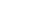causes an output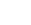and an input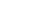causes an output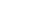, then an input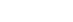gives an output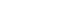. Hence, if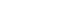then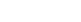.

If the rainfall excess in a duration D hours is r times the unit depth, the ordinates of the resulting DRH will be r times the corresponding ordinates of the D hour unit hydrograph. Since the area under the D hour DRH should be r times the area under the corresponding D hour unit hydrograph, the base of the DRH will be the same as that of the unit hydrograph.

The assumption of linear response enables the use of the method of superposition to derive DRHs.

If two rainfall excess of D hour duration each occur consecutively, their combined effect (ie. the resulting DRH) is obtained by superposing the respective DRHs, care being taken to account for proper sequence of events

#### Application of Unit Hydrograph

1. The DRH in a catchment due to a given storm can be determined if an appropriate unit hydrograph is available

2. Let it be assumed that D-h unit hydrograph and the storm hyetograph are available

3. The initial losses and infiltration losses are estimated and deducted from the storm hyetograph to obtain ERH

4. The ERH is then divided into M blocks of D-h duration each

5. The rainfall excess in each D-h duration is then operated upon the unit hydrograph successively to get various DRH curves. The ordinates of these DRHs are suitably lagged to obtain the proper time sequence and are then added at each time interval to obtain the required net DRH due to the storm

Consider a sequence of M rainfall excess values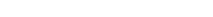each of duration D hours. u(t) is the ordinate of a D-h unit hydrograph at t hours from the beginning.• The direct runoff due to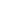at a time t is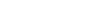The direct runoff due to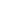at a time (t-D) is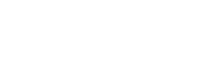Thus at any time t, the total direct runoff is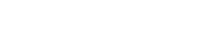• After deriving the net DRH, the estimated base flow is added to obtain the total flood hydrograph